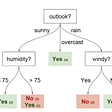# Confusion Matrix — Not So Confusing!

A Confusion Matrix is a performance measurement technique for Machine learning classification. It is a kind of table that helps you to know the performance of the classification model on a set of test data for that the true values are known. The term confusion matrix itself is very simple, but its related terminology can be a little confusing. Here, some simple explanation is given for this technique.

# Understanding TP, TN, FP & FN in a Confusion Matrix

• TP: True Positive: Predicted values correctly predicted as actual positive
• FP: Predicted values incorrectly predicted an actual positive. i.e., Negative values predicted as positive
• FN: False Negative: Positive values predicted as negative
• TN: True Negative: Predicted values correctly predicted as an actual negative

You can compute the accuracy-test from the confusion matrix:

# Example of Confusion Matrix:

Confusion Matrix is a useful machine learning method that allows you to measure Recall, Precision, Accuracy, and AUC-ROC curve. Below given is an example to know the terms True Positive, True Negative, False Negative, and True Negative.

True Positive:

You projected positive and it turns out to be true. For example, you had predicted that France would win the world cup, and it won.

True Negative:

When you predicted negative, and it’s true. You had predicted that England would not win and it lost.

False Positive:

Your prediction is positive, and it is false.

You had predicted that England would win, but it lost.

False Negative:

Your prediction is negative, and the result is also false.

You had predicted that France would not win, but it won.

# Precision vs. Recall

Precision tells us how many of the correctly predicted cases actually turned out to be positive.

Here’s how to calculate Precision:

This would determine whether our model is reliable or not.

Recall tells us how many of the actual positive cases we were able to predict correctly with our model.

And here’s how we can calculate Recall:

# F1-Score

In practice, when we try to increase the precision of our model, the recall goes down, and vice-versa. The F1-score captures both the trends in a single value:

F1-score is a harmonic mean of Precision and Recall, and so it gives a combined idea about these two metrics. It is maximum when Precision is equal to Recall.

I hope readers got a basic understanding of the confusion matrix and got to know how the confusion matrix works.

--

--

--

## More from Jane Alam

I am Data Scientist working in Cognizant | Writing about Data Science, AI, ML, DL, Stats, Math

Love podcasts or audiobooks? Learn on the go with our new app.

## Convolutional Neural Networks- Deep Learning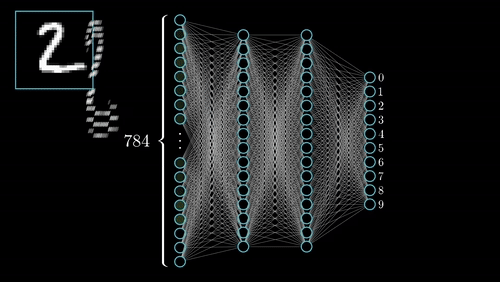## Decision Tree## OpenAI + ROS ?## Preventing imbalanced data from leading to abominable classification## Simple segmentation in computer vision## Explain like a FIVE: Deep Learning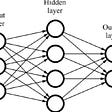## Reinforcement Learning: Learn from your mistakes## Machine Learning Basics with Examples — Part 1 Introduction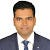## Jane Alam

I am Data Scientist working in Cognizant | Writing about Data Science, AI, ML, DL, Stats, Math

## LASSO (L1) Vs Ridge (L2) Vs Elastic Net Regularization For Classification Model## SF Crime prediction- A multi-class classification problem.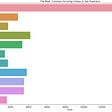## For Machine Learning Rookies-## A Layman’s guide to the internal of decision tree & random forest